• 匹配滤波器的一种实现，供初学者参考。有注释，方便理解。
• 匹配滤波器vhdl代码 滤波器 匹配滤波器vhdl代码 滤波器
• 匹配滤波器入门简介，介绍了关于匹配滤波器的基本知识，匹配滤波原理以及匹配方法
• 认知无线电有能量检测 匹配滤波器检测 周期循环平稳特征检测 仿真认知无线电中的匹配滤波器检测
• ## 匹配滤波器及matlab仿真

千次阅读 多人点赞 2020-06-03 15:28:15
匹配滤波器的性质3.1匹配滤波器的最大峰值信噪比3.2匹配滤波器的幅频特性相频特性3.3匹配滤波器的物理可实现性3.4输出信号和噪声3.5匹配滤波器的时延适应性3.6匹配滤波器的频移不适应性3.7输出信号频谱与输入信号...
随机信号处理笔记：匹配滤波器

——南京理工大学顾红老师的《随机信号处理》浅析

文章目录

随机信号处理笔记：匹配滤波器

1.线性滤波器输出端信噪比
2.匹配滤波器的传输函数和冲激响应

2.1复函数的施瓦兹不等式
2.2传输函数求解

3.匹配滤波器的性质

3.1匹配滤波器的最大峰值信噪比
3.2匹配滤波器的幅频特性相频特性
3.3匹配滤波器的物理可实现性
3.4输出信号和噪声
3.5匹配滤波器的时延适应性
3.6匹配滤波器的频移不适应性
3.7输出信号频谱与输入信号频谱关系

4.匹配滤波器的信号处理SNR增益

4.1matlab仿真匹配滤波增益

4.1.1理论值：
4.1.2仿真图片：


展开全文matlab
• 包含代码和说明文档，通过匹配滤波器实现图形识别，可用于匹配滤波器的学习
• 关于匹配滤波器的原理以及其matlab实现方式的详细过程 关于匹配滤波器的原理以及其matlab实现方式的详细过程
• 一种基于VHDL的数字匹配滤波器设计代码一种基于VHDL的数字匹配滤波器设计代码
• 关于匹配滤波器的原理以及其matlab实现方式的详细过程 匹配滤波器的简单实现
• 信号检测实验匹配滤波器信号检测实验匹配滤波器信号检测实验匹配滤波器
• 匹配滤波器的实验，带MATLAB程序，雷达LFM应用
• LFM雷达匹配滤波器仿真，针对线性调频波设计的匹配滤波器，亲测可用，适合初学者。
• 本文是关于移动通信中关于接受通道的匹配滤波器的FPGA实现
• 从频域直观理解匹配滤波器的效果(2) 匹配滤波器的冲激响应(3) 匹配滤波器的输出信噪比(4) 匹配滤波器的时间适应性(5) 匹配滤波器与自相关(6) 匹配滤波器进行频域滤波 参考 通信之道——从微积分到5G 匹配滤波器...


文章目录
(1) 匹配滤波器的公式推导与解释1.使用匹配滤波器的目的2.推导匹配滤波器的公式3.从频域直观理解匹配滤波器的效果
(2) 匹配滤波器的冲激响应(3) 匹配滤波器的输出信噪比(4) 匹配滤波器的时间适应性(5) 匹配滤波器与自相关(6) 匹配滤波器进行频域滤波

参考
通信之道——从微积分到5G匹配滤波器及其在雷达信号处理中的应用随机过程概论（PPT） 第四章线性调频信号
(1) 匹配滤波器的公式推导与解释
解释实际上是要分三步进行：首先要说明要用匹配滤波器干了一个什么事，其次要通过匹配滤波器要做的事说明为什么匹配滤波器的公式是这个形式，最后又要从直观的角度来说明匹配滤波器的这个公式为什么能让它做成这个事情。
1.使用匹配滤波器的目的
当有用信号通过信道传输后，混杂有许多高斯白噪声，信号被噪声淹没。通过匹配滤波器提高信噪比，使有用信号在时域上更加明显，更易被检测到。
2.推导匹配滤波器的公式
推导公式从匹配滤波器要实现的功能出发：提高信噪比。一个实信号

s

(

t

)

s(t)

通过 AWGN 信道，得到

r

(

t

)

=

s

(

t

)

+

n

(

t

)

r(t) = s(t) + n(t)

。其中

n

(

t

)

n(t)

是高斯白噪声。同时已知的高斯白噪声的相关函数

R

(

τ

)

=

N

0

2

δ

(

τ

)

R(\tau) = \displaystyle\frac{N_0}{2}\delta(\tau)

让被污染的信号通过一个时域冲激响应为

h

(

t

)

h(t)

的系统并在

t

0

t_0

时刻采样可以得到

y

(

t

0

)

=

∫

−

∞

∞

r

(

τ

)

h

(

t

0

−

τ

)

d

τ

=

∫

−

∞

∞

s

(

τ

)

h

(

t

0

−

τ

)

d

τ

+

∫

−

∞

∞

n

(

t

)

h

(

t

0

−

t

)

d

t

=

y

s

(

t

0

)

+

y

n

(

t

0

)

\begin{aligned} y(t_0) &= \int_{-\infin}^{\infin}r(\tau)h(t_0-\tau)d\tau\\ &=\int_{-\infin}^{\infin}s(\tau)h(t_0-\tau)d\tau + \int_{-\infin}^{\infin}n(t)h(t_0-t)dt\\ &=y_s(t_0) + y_n(t_0) \end{aligned}

很容易发现，

y

s

(

t

0

)

y_s(t_0)

是有用信号，

y

n

(

t

0

)

y_n(t_0)

是噪声信号，由此定义信噪比

S

N

R

=

y

s

2

(

t

0

)

E

[

y

n

2

(

t

0

)

]

SNR = \displaystyle\frac{y_s^2(t_0)}{E[y_n^2(t_0)]}

同时可以推导出

E

[

y

n

2

(

t

0

)

]

E[y_n^2(t_0)]

的表达式

E

[

y

n

2

(

t

0

)

]

=

E

[

∫

−

∞

∞

n

(

τ

)

h

(

t

0

−

τ

)

d

τ

∫

−

∞

∞

n

(

t

)

h

(

t

0

−

t

)

d

t

]

=

∫

−

∞

∞

∫

−

∞

∞

N

0

2

δ

(

t

−

τ

)

h

(

t

0

−

τ

)

h

(

t

0

−

t

)

d

τ

d

t

=

N

0

2

∫

−

∞

∞

h

2

(

t

0

−

τ

)

d

τ

\begin{aligned} E[y_n^2(t_0)] &= E[\int_{-\infin}^{\infin}n(\tau)h(t_0-\tau)d\tau\int_{-\infin}^{\infin}n(t)h(t_0-t)dt]\\ &=\int_{-\infin}^{\infin}\int_{-\infin}^{\infin}\frac{N_0}{2}\delta(t-\tau)h(t_0-\tau)h(t_0-t) d\tau dt\\ &=\frac{N_0}{2}\int_{-\infin}^{\infin}h^2(t_0-\tau) d\tau \end{aligned}

回代并求出最大值

S

N

R

=

2

[

∫

−

∞

∞

s

(

τ

)

h

(

t

0

−

τ

)

d

τ

]

2

N

0

∫

−

∞

∞

h

2

(

t

0

−

τ

)

d

τ

≤

2

∫

−

∞

∞

s

2

(

τ

)

d

τ

∫

−

∞

∞

h

2

(

t

0

−

τ

)

d

τ

N

0

∫

−

∞

∞

h

2

(

t

0

−

τ

)

d

τ

=

2

N

0

∫

−

∞

∞

s

2

(

τ

)

d

τ

\begin{aligned} SNR&=\displaystyle\frac{2[\displaystyle\int_{-\infin}^{\infin}s(\tau)h(t_0-\tau)d\tau]^2}{N_0\displaystyle\int_{-\infin}^{\infin}h^2(t_0-\tau)d\tau}\le \displaystyle\frac{2\displaystyle\int_{-\infin}^{\infin}s^2(\tau)d\tau\displaystyle\int _{-\infin}^{\infin}h^2(t_0-\tau)d\tau}{N_0\displaystyle\int_{-\infin}^{\infin}h^2(t_0-\tau)d\tau}\\ &=\displaystyle\frac{2}{N_0}\displaystyle\int_{-\infin}^{\infin}s^2(\tau)d\tau \end{aligned}

上面的式子在放缩的过程中使用了柯西不等式，所以想要达到最大值就需要根据柯西不等式等号成立的条件得到

h

(

t

0

−

τ

)

=

s

(

τ

)

⇒

h

(

t

)

=

k

s

(

t

0

−

t

)

h(t_0-\tau) = s(\tau)\Rightarrow h(t) = ks(t_0-t)

3.从频域直观理解匹配滤波器的效果
由时域表达式可以推出频域表达式

H

(

ω

)

=

k

S

∗

(

ω

)

e

−

j

ω

t

0

H(\omega) = kS^*(\omega)e^{-j\omega t_0}

，可以发现有

∣

H

(

ω

)

∣

=

k

∣

S

(

ω

)

∣

|H(\omega)| = k|S(\omega)|

，也就是说系统的增益和信号的幅度谱是一样的。由于白噪声的频谱是常数，就是说在哪里都一样，所以系统在信号幅度谱更大的地方（含有的有用信号会更多）使用更大的增益就可以让有用信号在总的信号中增长的更多，也就是说提高了信噪比。
(2) 匹配滤波器的冲激响应
由之前的推证可以知道，冲激响应的时域表达式为

h

(

t

)

=

k

s

(

t

0

−

t

)

h(t) = ks(t_0-t)

。  注意

h

(

t

)

h(t)

与

t

0

t_0

值选取需要遵循的准则： 因果关系

h

(

t

)

=

{

s

(

t

0

−

t

)

,

t

≥

0

0

,

t

<

0

h(t) = \begin{cases} s(t_0-t),t\ge 0\\ 0,t<0 \end{cases}

为了使输入信号的全部都能对输出信号有所贡献，采样时刻

t

0

t_0

应满足

s

(

t

)

=

0

,

t

>

t

0

s(t)=0,t>t_0

。比如信号产生的时间是

[

0

,

10

μ

s

]

[0,10\mu s]

，采样时刻

t

0

t_0

就至少是

10

μ

s

10\mu s

。  这里假定采样时刻 T = 12

μ

\mu

s，信号带宽 B = 20MHz，信号时宽 TimeWidth = 10

μ

\mu

s，信号的采样频率

F

s

F_s

= 50MHz，起始频率为 0Hz。  matlab 代码 %% 产生线性调频波
TimeWidth = 10e-6;      %脉冲持续时间10us
T = 8e-6;               %T在8us
BandWidth = 20e6;       %线性调频信号的频带宽度20MHz
Fs = 50e6;              %采样频率，注意需要满足奈奎斯特频率
sample_dot_num = round(TimeWidth * Fs);%表示采样点的个数

f0 = 0;%初始频率
f1 = f0 + BandWidth;%终止频率
t=0:1/Fs:TimeWidth;%根据结束时间生成时间序列
signal = chirp_signal(t,f0,f1);
plot(t*1e6,signal,'LineWidth',2);
%% 匹配滤波器的脉冲响应
%这里 k 假定取 1
h = signal(end:-1:1);
t = -t(end:-1:1) + T;
plot(t*1e6,h,'LineWidth',2);
  仿真结果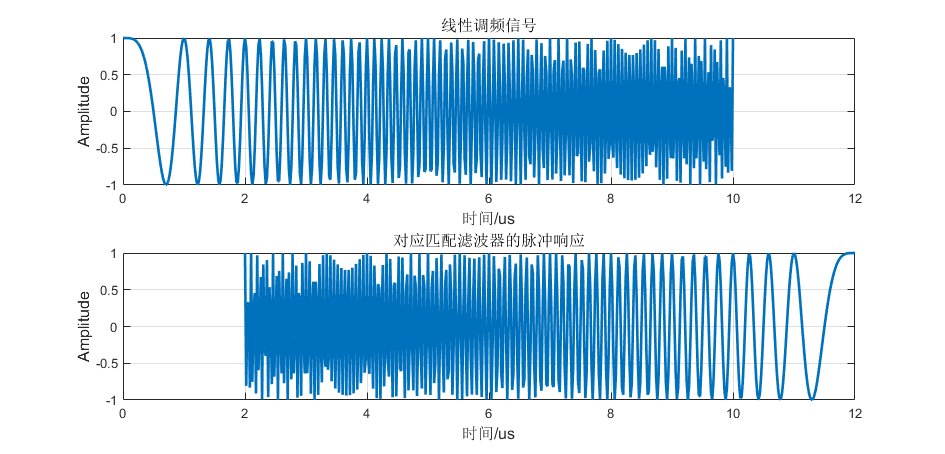(3) 匹配滤波器的输出信噪比
匹配滤波器的输出功率信噪比只取决于输入信号的能量和白噪声功率谱密度而与输入信号形状和噪声分布规律无关。  这里在仿真时均假定 snr=0，并且通过增添频率移动量保证整体能量基本相等，均为

125.5

125.5

左右。  matlab 代码： %% 产生线性调频信号
TimeWidth = 10e-6;      %脉冲持续时间10us
BandWidth = 20e6;       %线性调频信号的频带宽度20MHz
Fs = 50e6;Ts = 1/Fs;    %采样频率，注意需要满足奈奎斯特频率
N = fix(TimeWidth/Ts);
f0 = 0;%初始频率
f1 = f0 + BandWidth;%终止频率

%设定频率偏移量
f_shifta = 5e6;
f_shiftb = 10e6;
t=0:1/Fs:TimeWidth;%根据结束时间生成时间序列
%采用不同的频率偏移量生成两个能量（几乎）相同的信号
signal1 = chirp_signal(t,f0+f_shifta,f1);
signal2 = chirp_signal(t,f0+f_shiftb,f1);
plot(t*1e6,signal1);
plot(t*1e6,signal2);
%% 加入噪声后
%加入噪声
snr = 0;
y1 = awgn(signal1, snr, 'measured');
y2 = awgn(signal2, snr, 'measured');
plot(t*1e6,y1);
plot(t*1e6,y2);
% 经过匹配滤波器
mf=fliplr(signal1);     %输入序列取反序（匹配滤波器的脉冲响应）
sr_noise_mf=conv(mf,y1); %有噪声回波信号匹配滤波（使用脉冲响应和输入信号进行卷积）
plot((0:length(sr_noise_mf)-1)/Fs*1e6,20*log10(abs(sr_noise_mf)))
mf=fliplr(signal2);     %输入序列的复共轭（匹配滤波器的脉冲响应）
sr_noise_mf=conv(mf,y2); %有噪声回波信号匹配滤波（使用脉冲响应和输入信号进行卷积）
plot((0:length(sr_noise_mf)-1)/Fs*1e6,20*log10(abs(sr_noise_mf)))
  仿真结果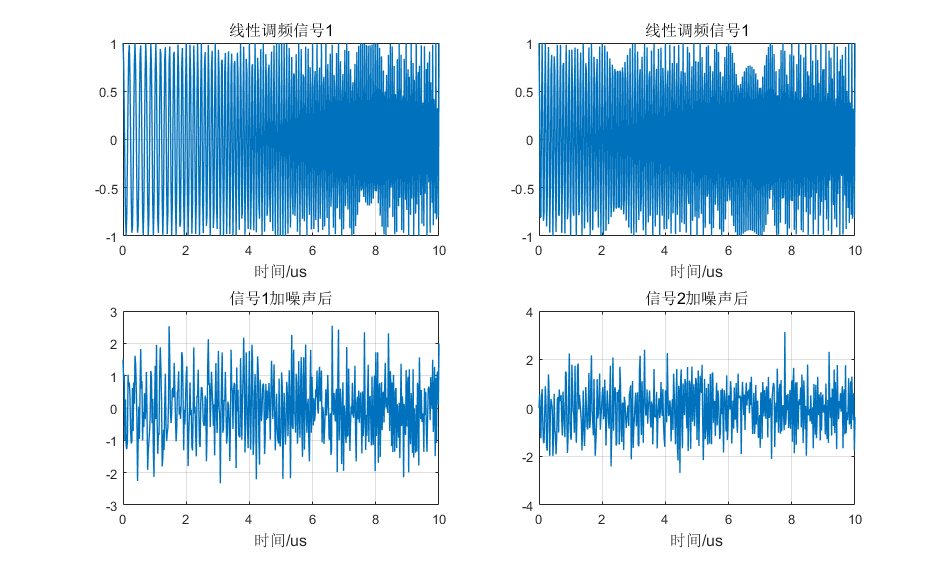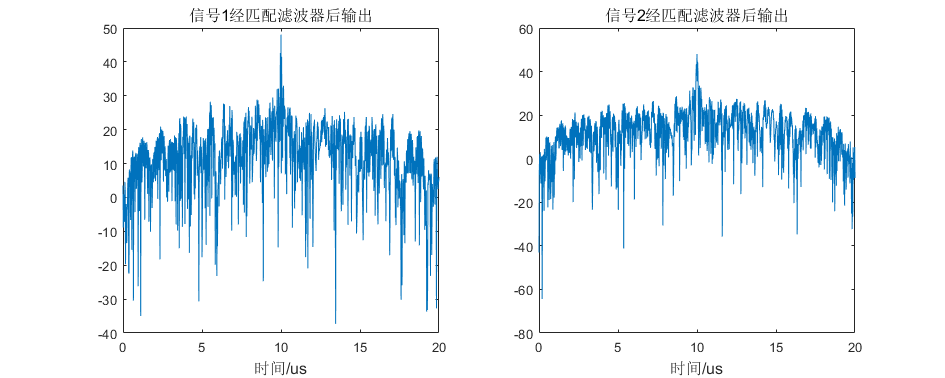可以看到在信号能量基本相等的情况下，通过匹配滤波器后的信噪比最大的位置基本相同。
(4) 匹配滤波器的时间适应性
匹配滤波器对振幅和时延参量不同的信号具有适应性，具体来说就是与信号

s

(

t

)

s(t)

相匹配的滤波器的系统函数

H

(

ω

)

H(\omega)

，对于信号

s

1

(

t

)

=

A

s

(

t

−

τ

)

s_1(t) = As(t-\tau)

来说也是匹配的，只不过最大输出功率信噪比出现的时刻延迟了

τ

\tau

。matlab 代码%% 产生线性调频信号
TimeWidth = 10e-6;      %脉冲持续时间10us
TimeEmpty = 20e-6;
BandWidth = 20e6;       %线性调频信号的频带宽度20MHz
Fs = 50e6;Ts = 1/Fs;    %采样频率，注意需要满足奈奎斯特频率
N = fix(TimeWidth/Ts);

f0 = 0;%初始频率
f1 = f0 + BandWidth;%终止频率
t=0:1/Fs:TimeWidth;%根据结束时间生成时间序列
signal = chirp_signal(t,f0,f1);
t_total = linspace(0,2*(TimeEmpty+TimeWidth),2*(TimeEmpty+TimeWidth)/Ts);
signal_total = [signal(1:end-1),zeros(1,fix(TimeEmpty/Ts)),signal(1:end-1),zeros(1,fix(TimeEmpty/Ts))];
plot(t_total*1e6,signal_total,'LineWidth',2);
%% 加入噪声后
%加入噪声
snr = 0;
signal_with_noise = awgn(signal_total, snr, 'measured');
plot(t_total*1e6,signal_with_noise,'LineWidth',2);
%% 经过匹配滤波器后(信号波形通过与其对应的匹配滤波器)
%这里 k 假定取 1
h=zeros(1,N);
t1 = fix(TimeWidth/Ts);
for tt=0:N-1
h(tt+1)=signal_total(t1-tt);%匹配滤波器
end
% 经过匹配滤波器
sr_noise_mf=conv(h,signal_with_noise); %有噪声回波信号匹配滤波（使用脉冲响应和输入信号进行卷积）
plot((0:length(sr_noise_mf)-1)/Fs*1e6,20*log10(abs(sr_noise_mf)),'LineWidth',2);
 仿真结果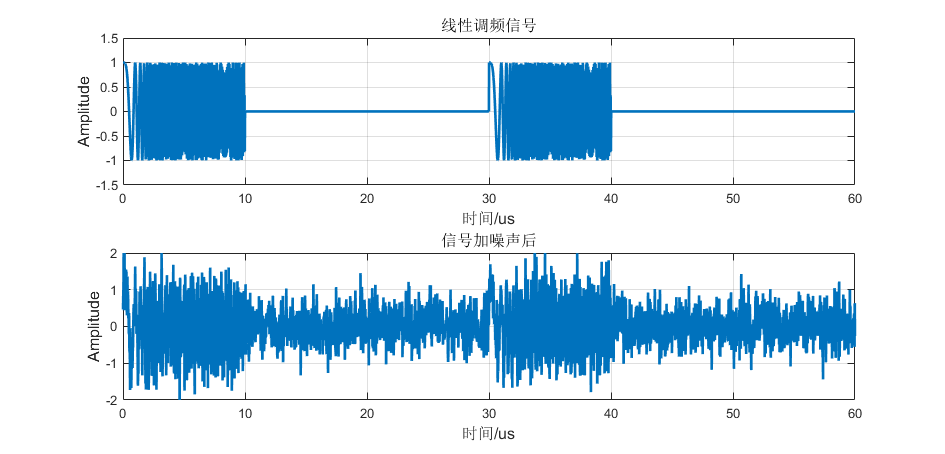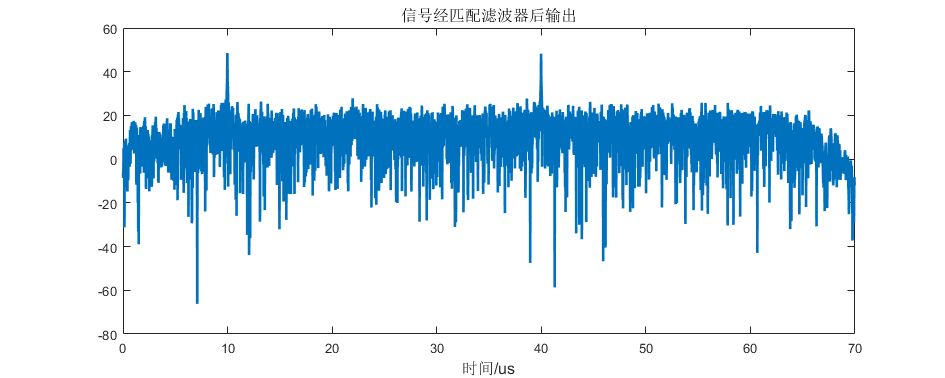可以看出来，对于有一个时延的信号，使用相同的匹配滤波器会导致输出在信噪比最大的地方也有一个响应的时延。
(5) 匹配滤波器与自相关
matlab代码%% 产生线性调频信号
TimeWidth = 10e-6;      %脉冲持续时间10us
BandWidth = 20e6;       %线性调频信号的频带宽度20MHz
Fs = 50e6;Ts = 1/Fs;    %采样频率，注意需要满足奈奎斯特频率
N = fix(TimeWidth/Ts);
f0 = 0;%初始频率
f1 = f0 + BandWidth;%终止频率
t=0:1/Fs:TimeWidth;%根据结束时间生成时间序列
signal = chirp_signal(t,f0,f1);
signal = awgn(signal,0,'measured');%这里添加白噪声
plot(t*1e6,signal,'LineWidth',2);
%% 匹配滤波
%计算匹配滤波器的冲激响应函数
h = zeros(1,N);
t1 = fix(TimeWidth/Ts);
for tt=0:N-1
h(tt+1) = signal(t1-tt);%匹配滤波器
end
signal_match = abs(conv(h,signal));
t_match = (0:length(signal_match)-1)/Fs;
plot(t_match*1e6,signal_match,'LineWidth',2);
%% 求自相关函数
signal_autocorrelation = abs(xcorr(signal));
t_autocorrelation = (0:length(signal_autocorrelation)-1)/Fs;
plot(t_autocorrelation*1e6,signal_autocorrelation,'LineWidth',2);
 仿真结果：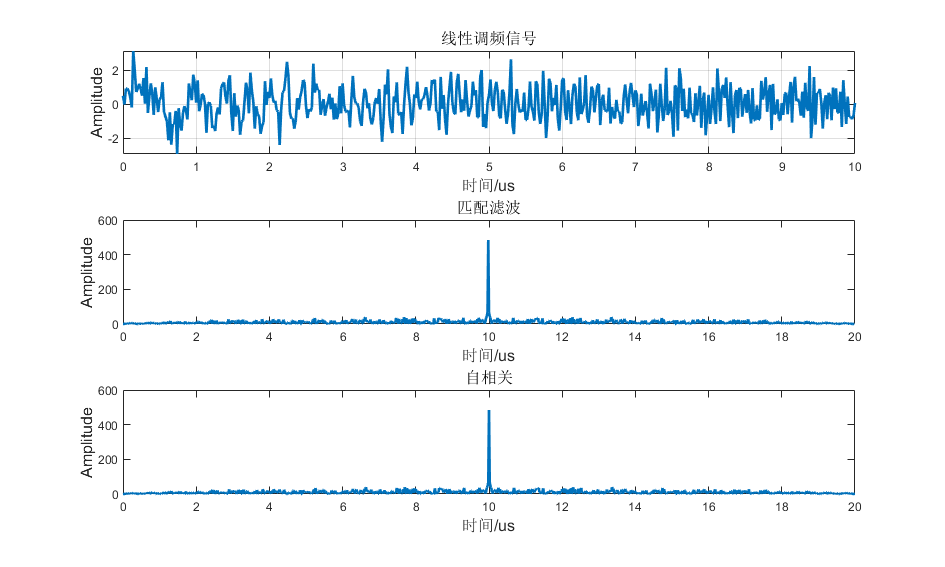从波形上来看两者是完全相同的，但是一定要注意前提条件。在

t

=

t

0

t=t_0

并且噪声

n

(

t

)

n(t)

为零均值白噪声的条件下匹配滤波器与相关器的输出是相等的。
(6) 匹配滤波器进行频域滤波
matlab 代码 %% 产生线性调频信号
TimeWidth = 10e-6;      %脉冲持续时间10us
BandWidth = 20e6;       %线性调频信号的频带宽度20MHz
Fs = 50e6;Ts = 1/Fs;    %采样频率，注意需要满足奈奎斯特频率
N = fix(TimeWidth/Ts);
f0 = 0;%初始频率
f1 = f0 + BandWidth;%终止频率
t=0:1/Fs:TimeWidth;%根据结束时间生成时间序列
signal = chirp_signal(t,f0,f1);

snr = 0;
signal_with_noise = awgn(signal, snr, 'measured');  %回波信号实际上就是给原信号增加了噪声
plot(t*1e6,signal_with_noise,'LineWidth',2);

freq=linspace(0,Fs,N);
plot(freq*1e-6,fftshift(abs(fft(signal_with_noise(1:end-1)))),'LineWidth',2);

%% 进行匹配滤波
%计算匹配滤波器的冲激响应函数
h = zeros(1,N);
t1 = fix(TimeWidth/Ts);
for tt=0:N-1
h(tt+1) = signal(t1-tt);%匹配滤波器
end
subplot(311)
plot(freq*1e-6,abs(fftshift(fft(h)).* fftshift(fft(signal_with_noise(1:end-1)))),'LineWidth',2);

%% 求相关
temp = abs(ifft(fftshift(fft(h)).* fftshift(fft(signal_with_noise(1:end-1)))));
temp = [temp,temp(end:-1:1)];
plot((0:length(temp)-1)*Ts*1e6,temp,'LineWidth',2);
%% 求匹配
subplot(313)
temp = abs(conv(h,signal_with_noise(1:end-1)));
plot((0:length(temp)-1)*Ts*1e6,temp,'LineWidth',2);
  仿真结果：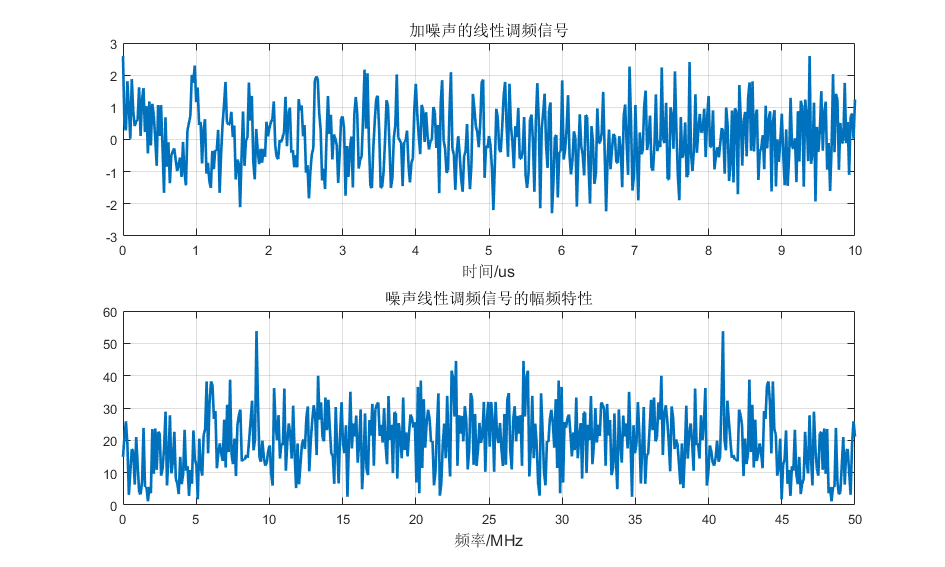对淹没在 0dB 高斯白噪声中的信号直接进行 FFT，可以看到在频域并不能很好的分出信号。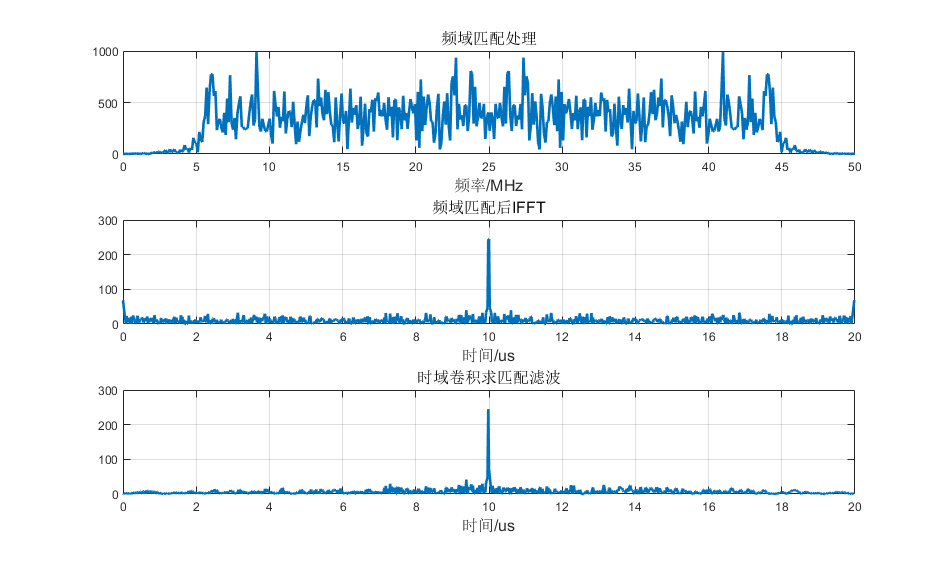频域乘积：对加噪信号和匹配滤波器脉冲响应分别进行 FFT 变换，得到两组频谱后点乘然后进行 IFFT 变换即可得出滤波结果。从时域卷积：让加噪信号和匹配滤波器进行卷积，直接得到结果，可以看得这两种操作使结果 基本相同。 
展开全文• 基于光学衍射理论,探讨了采用准单色光照明的条带模式合成孔径激光雷达(SAL)的成像过程,给出了几种情况下,方位合成孔径成像匹配滤波器的解析表达形式及相应的成像分辨率。结果表明,条带模式SAL方位成像匹配滤波器的...
• 多用户检测中匹配滤波器的FPGA实现，赵彦龙，李艳萍，首先简要介绍匹配滤波器在多用户检测中的作用，以及多用户检测匹配滤波器的基本结构和工作原理，在此基础上提出了一种基于FPGA(Fiel...